Tamilnadu State Board New Syllabus Samacheer Kalvi 12th Maths Guide Pdf Chapter 11 Probability Distributions Ex 11.5 Textbook Questions and Answers, Notes.

## Tamilnadu Samacheer Kalvi 12th Maths Solutions Chapter 11 Probability Distributions Ex 11.5

Question 1.
Compute P(X = k) for the binomial distribution, B(n, p) where
(i) n = 6, p = $$\frac { 1 }{ 3 }$$, k = 3
(ii) n = 10, p = $$\frac { 1 }{ 5 }$$, k = 4
(iii) n = 9, p = $$\frac { 1 }{ 2 }$$, k = 7
Solution: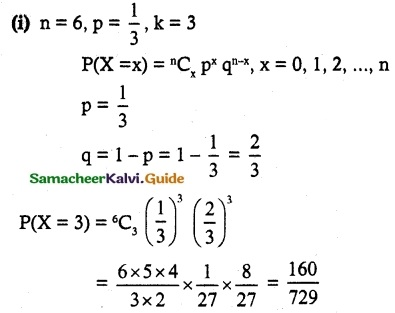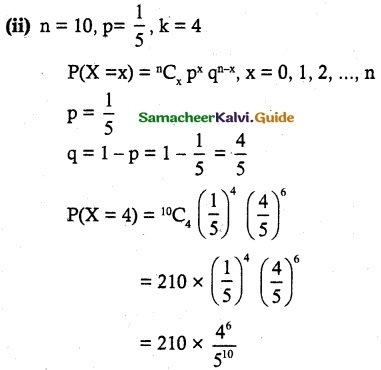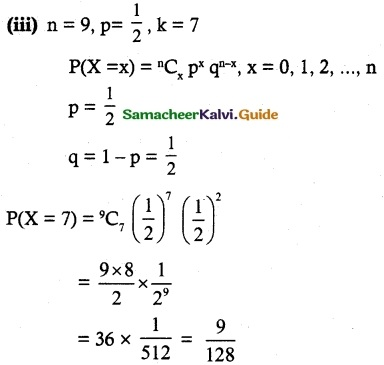Question 2.
The probability that Mr. Q hits a target ait any trial is $$\frac { 1 }{ 4 }$$. Suppose he tries at the target 10 times. Find the probability that he hits the target (i) exactly 4 times (ii) atleast one time
Solution:
Let p be the probability of hitting the target
n = 10, p = $$\frac { 1 }{ 4 }$$, X ~ B(n, p)
P(X = x) = ⁿCx px qn-x, x = 0, 1, 2, …. n
q = 1 – p = $$\frac { 3 }{ 4 }$$
(i) Probability of hitting the target exactly 4 times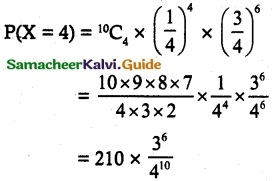(ii) Probability of hitting atleast one time
P(X ≥ 1) = 1 – P(X < 1) = 1 – P(X = 0)
= 1 – 10C0 × ($$\frac { 1 }{ 4 }$$)° × ($$\frac { 3 }{ 4 }$$)10
= 1 – $$\frac { 3^{10} }{ 4^{10} }$$Question 3.
Using binomial distribution find the mean and variance of X for the following experiments
(i) A fair coin is tossed 100 times and X denotes the number of heads.
(ii) A fair die is tossed 240 times and X denotes the number of times that four appeared.
Solution:
(i) Let p be the probability of getting heads
⇒ p = $$\frac { 1 }{ 2 }$$
n = 100, p = $$\frac { 1 }{ 2 }$$, X ~ B(n, p)
q = 1 – p = $$\frac { 1 }{ 2 }$$
Mean = np
= 100 × $$\frac { 1 }{ 2 }$$
= 50
Variance = npq
= 50 × $$\frac { 1 }{ 2 }$$
= 25

(ii) Let p be the probability of getting 4 when a die is thrown
n = 240, p = $$\frac { 1 }{ 6 }$$, X ~ B(n, p)
q = 1 – p = $$\frac { 5 }{ 6 }$$
Mean = np
= 240 × $$\frac { 1 }{ 6 }$$ = 40
Variance = npq
= 40 × $$\frac { 5 }{ 6 }$$ = $$\frac { 100}{ 3 }$$Question 4.
The probability that a certain kind of component will survive a electrical test is $$\frac { 3 }{ 4 }$$. Find the probability that exactly 3 of the 5 components tested survive.
Solution:
Let p be the probability of a certain component survive in an electrical test.
n = 5, p =$$\frac { 3 }{ 4 }$$, X ~ B(n, p), k = 3
q = 1 – p = $$\frac { 1 }{ 4 }$$
P(X = x) = ⁿCx px qn-x, x = 0, 1, 2, …. n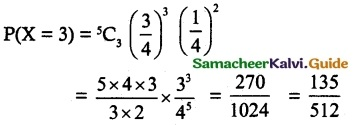Question 5.
A retailer purchases a certain kind of electronic, device from a manufacturer. The manufacturer indicates that the defective rate of the device 5 is 5%. The inspector of the retailer randomly picks 10 items from a shipment. What is the probability that there will be
(i) atleast one defective item
(ii) exactly two defective items?
Solution:
Let p be the probability that indicates the defective rate of an electronic device
p = 5%
= 0.05
q = 1 – p = 0.95
n = 10, p = 0.05, X ~ B(n, p)
P(X = x) = ⁿCx px qn-x, x = 0, 1, 2, …. n
(i) Atleast 1 defective item
P(X ≥ 1) = 1 – (X < 1)
= 1 – P(X = 0)
= 1 – 10C0 (0.05)° (0.95)10
= 1 – (0.95)10

(ii) Exactly two defective items
P(X = 2) = 10C2 (0.05)² (0.95)8Question 6.
If the probability that fluorescent light has a useful life of atleast 600 hours is 0.9, find the probabilities that among 12 such lights
(i) exactly 10 will have a useful life of atleast 600 hours
(ii) At atleast 11 will have a useful life of atleast 600 hours
(iii) At atleast 2 will not have a useful life of at least 600 hours.
Solution:
Let p be the probability of the useful life hours of a fluorescent light.
n = 12, p = 0.9, X ~ B(n, p)
q – 1 – p = 0.1
P(X = x) = ⁿCx px qn-x, x = 0, 1, 2, …. n
(i) Exactly 10
P(X = 10) = 12C10 (0.9)10 (0.1)²

(ii) Atleast 11
P(X ≥ 11) = P(X = 11) + P(X = 12)
= 12C11 (0.9)11 (0.1)1 + 12C12 (0.9)12 (0.1)°
= 12 × (0.9)11 × 0.1 + 1 × (0.9)12 × 1
= (0.9)11 (12 × 0.1 × 0.9)
= (0.9)11 (1.2 + 0.9)
= (2.1) (0.9)11

(iii) Atleast 2 will not have a useful
P(X ≤ 10) = 1 – P(X > 10)
= 1 – [P(X = 11) + P(X = 12)]
= 1 – (2.1)(0.9)11Question 7.
The mean and standard deviation of a binomial variate X are respectively 6 and 2. Find
(i) The probability mass function
(ii) P(X = 3)
(iii) P(X ≥ 2).
Solution:
X ~ B(n, p)
Given, Mean = 6, Standard deviation = 2
np = 6, $$\sqrt { npq }$$ = 2
npq = 4
$$\frac { npq }{ np }$$ = $$\frac { 4 }{ 6 }$$
q = $$\frac { 2 }{ 3 }$$
p = 1 – q = $$\frac { 1 }{ 3 }$$
npq = 4
n × $$\frac { 1 }{ 3 }$$ × $$\frac { 2 }{ 3 }$$ = 4
n = 18
(i) Probability mass function
P(X = x) = ⁿCx px qn-x, x = 0, 1, 2, …. n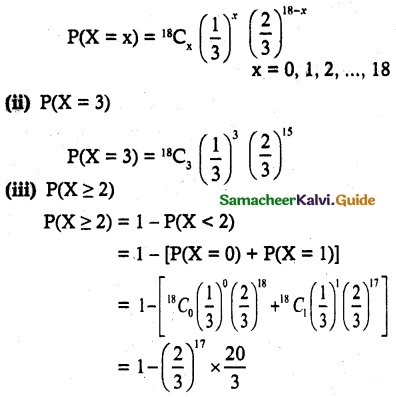Question 8.
If X ~ B(n, p) such that 4P(X = 4) = P(x = 2) and n = 6. Find the distribution, mean and standard deviation of X.
Solution:
X ~ B(n, p)
Given, 4P(X = 4) = P(X = 2), n = 6
P(X = x) = ⁿCx px qn-x, x = 0, 1, 2, …. n
⇒ 4 6C4 p4 q² = 6C2 p² q4
⇒ 4 p² = q²
4 (1 – q)² = q²
4(1 – 2q + q²) = q²
3q² – 8q + 4 = 0
(q – 2) (3q – 2) = 0
q = $$\frac { 2 }{ 3 }$$ (q ≠ 2)
Distribution
P(X = x) = 6Cx ($$\frac { 1 }{ 3 }$$)x ($$\frac { 2 }{ 3 }$$)6-x
x = 0, 1, 2, 3, 4, 5, 6
Mean = np = 6 × $$\frac { 1 }{ 3 }$$ = 2
Variance = npq = 2 × $$\frac { 2 }{ 3 }$$ = $$\frac { 4 }{ 3 }$$Question 9.
In a binomial distribution consisting of 5 independent trials, the probability of 1 and 2 successes are 0.4096 and 0.2048 respectively. Find the mean and variance of the random variable.
Solution:
n = 5, X ~ B(n, p)
Given, P(X = 1) = 0.4096
P(X = 2) = 0.2048
P(X = x) = ⁿCx px qn-x, x = 0, 1, 2, …. n
5C1 p q4 = 0.4096
5C2 p² q³ = 0.2048
5 p q4 = 0.4096 …….. (1)
10 p² q³ = 0.2048 ………. (2)
(1) divided by (2),
⇒ $$\frac { 5pq^4 }{ 10p^2q^3 }$$ = 2
$$\frac { p }{ q }$$ = 4
q = 4p
q = 4 (1 – q)
q = 4 – 4q
5q = 4
q = $$\frac { 4 }{ 5 }$$
p = 1 – q = $$\frac { 1 }{ 5 }$$
Mean = np
= 5 × $$\frac { 1 }{ 5 }$$ = 1
Variance = npq
= 1 × $$\frac { 4 }{ 5 }$$ = $$\frac { 4 }{ 5 }$$
Distribution
P(X = x) = 5Cx ($$\frac { 1 }{ 5 }$$)x ($$\frac { 4 }{ 5 }$$)5-x
x = 0, 1, 2, 3, 4, 5.